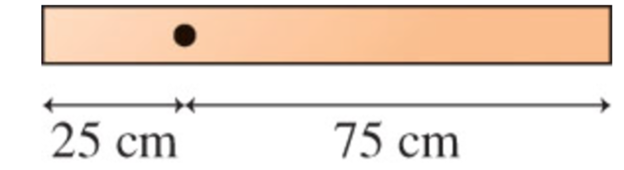# Problem: The 4.0 kg, uniform, horizontal rod in(Figure 1) is seen from the side.What is the gravitational torque about the point shown?

###### FREE Expert Solution

Torque:

$\overline{){\mathbf{\tau }}{\mathbf{=}}{\mathbf{r}}{\mathbf{F}}}$

The weight is acting at 50 cm. The rod will be made to experience a clockwise torque.

79% (34 ratings)###### Problem Details

The 4.0 kg, uniform, horizontal rod in(Figure 1) is seen from the side.What is the gravitational torque about the point shown?